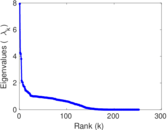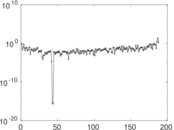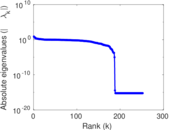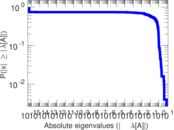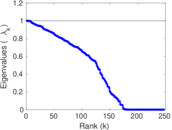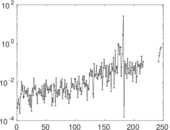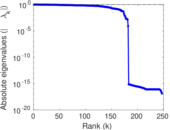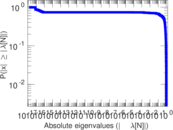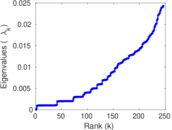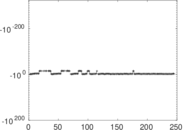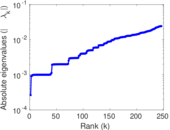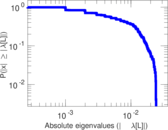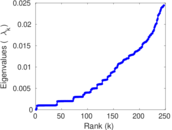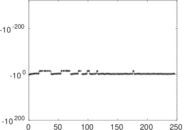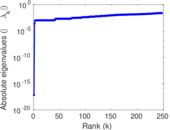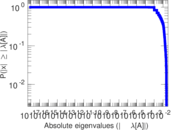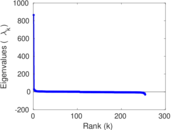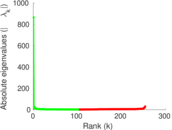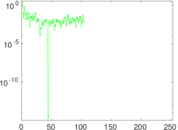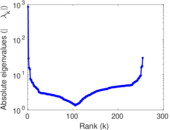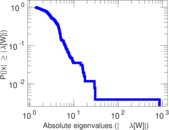# Unicode languages

This bipartite network denotes which languages are spoken in which countries. Nodes are countries and languages; edge weights denote the proportion (between zero and one) of the population of a given country speaking a given language. To quote the Unicode data description: "The main goal is to provide approximate figures for the literate, functional population for each language in each territory: that is, the population that is able to read and write each language, and is comfortable enough to use it with computers."

 Code `UL` Internal name `unicodelang` Name Unicode languages Data source http://www.unicode.org/cldr/charts/25/supplemental/territory_language_information.html AvailabilityDataset is available for download Consistency checkDataset passed all tests Category Feature network Dataset timestamp 2015 Node meaning Country, language Edge meaning Hosts Network formatBipartite, undirected Edge type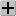Positive weights, no multiple edges Zero weights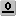Edges may have weight zero

## Statistics

 Size n = 868 Left size n1 = 254 Right size n2 = 614 Volume m = 1,255 Wedge count s = 21,977 Claw count z = 521,909 Cross count x = 15,999,004 Square count q = 1,266 4-Tour count T4 = 86,712 Maximum degree dmax = 141 Maximum left degree d1max = 69 Maximum right degree d2max = 141 Average degree d = 2.891 71 Average left degree d1 = 4.940 94 Average right degree d2 = 2.043 97 Fill p = 0.007 091 74 Size of LCC N = 858 Diameter δ = 8 50-Percentile effective diameter δ0.5 = 3.510 60 90-Percentile effective diameter δ0.9 = 5.243 55 Median distance δM = 4 Mean distance δm = 4.075 74 Gini coefficient G = 0.583 143 Balanced inequality ratio P = 0.269 439 Left balanced inequality ratio P1 = 0.315 552 Right balanced inequality ratio P2 = 0.321 881 Relative edge distribution entropy Her = 0.889 358 Power law exponent γ = 2.865 13 Tail power law exponent γt = 2.371 00 Tail power law exponent with p γ3 = 2.371 00 p-value p = 0.122 000 Left tail power law exponent with p γ3,1 = 2.371 00 Left p-value p1 = 0.075 000 0 Right tail power law exponent with p γ3,2 = 2.521 00 Right p-value p2 = 0.007 000 00 Degree assortativity ρ = −0.251 443 Degree assortativity p-value pρ = 2.076 20 × 10−17 Spectral norm α = 7.934 29 Algebraic connectivity a = 0.000 263 914 Spectral separation |λ1[A] / λ2[A]| = 1.862 52 Controllability C = 408 Relative controllability Cr = 0.521 739

## Plots

### Fruchterman–Reingold graph drawing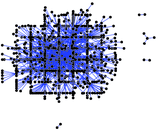### Degree distribution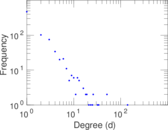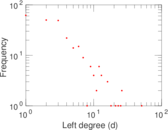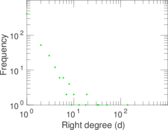### Cumulative degree distribution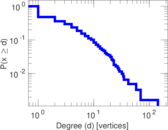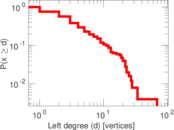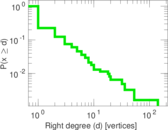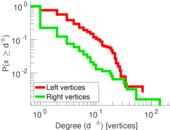### Lorenz curve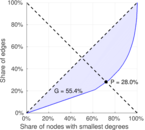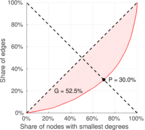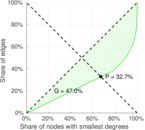### Spectral distribution of the adjacency matrix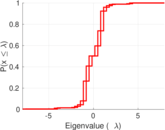### Spectral distribution of the normalized adjacency matrix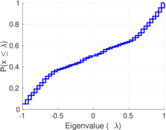### Spectral distribution of the Laplacian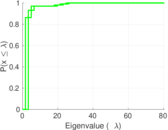### Spectral graph drawing based on the adjacency matrix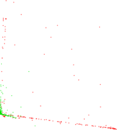### Spectral graph drawing based on the Laplacian### Spectral graph drawing based on the normalized adjacency matrix### Degree assortativity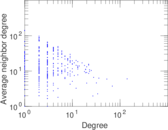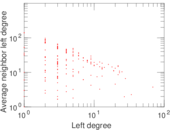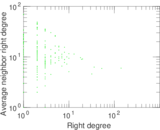### Zipf plot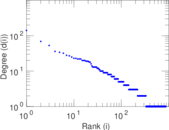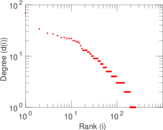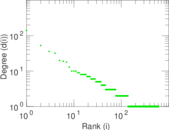### Hop distribution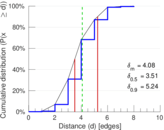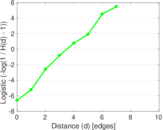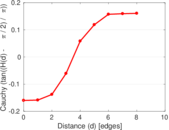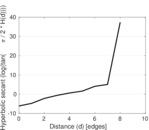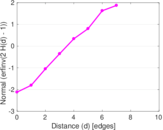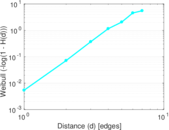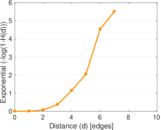### Double Laplacian graph drawing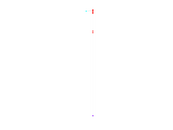### Delaunay graph drawing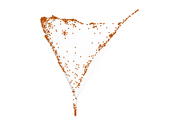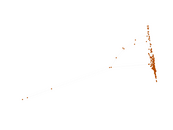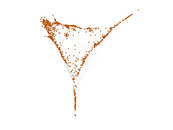### Edge weight/multiplicity distribution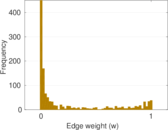### Matrix decompositions plots